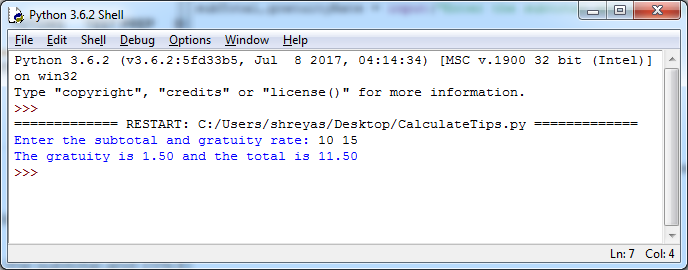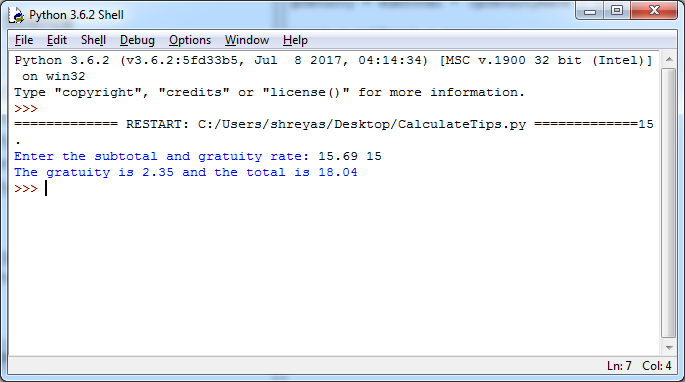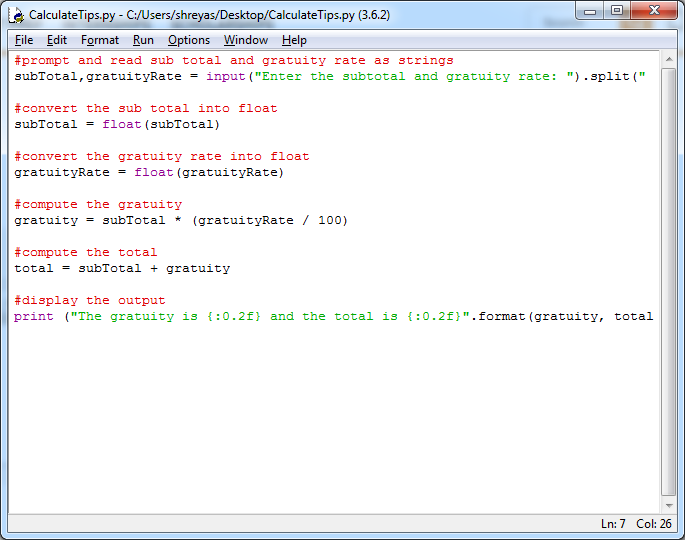# Question & Answer: PYTHON PROGRAMMING…..

PYTHON PROGRAMMING

(Financial application: calculate tips) Write a program that reads the subtotal and the gratuity rate and computes the gratuity and total.

Don't use plagiarized sources. Get Your Custom Essay on
GET AN ESSAY WRITTEN FOR YOU FROM AS LOW AS \$13/PAGE

For example, if the user enters 10 for the subtotal and 15% for the gratuity rate, the program displays 1.5 as the gratuity and 11.5 as the total.

Here is a sample run:

Enter the subtotal and a gratuity rate:
The gratuity is 2.35 and the total is 18.04

Program:

#prompt and read sub total and gratuity rate as strings
subTotal,gratuityRate = input(“Enter the subtotal and gratuity rate: “).split(” “)

#convert the sub total into float
subTotal = float(subTotal)

#convert the gratuity rate into float
gratuityRate = float(gratuityRate)

#compute the gratuity
gratuity = subTotal * (gratuityRate / 100)

#compute the total
total = subTotal + gratuity

#display the output
print (“The gratuity is {:0.2f} and the total is {:0.2f}”.format(gratuity, total))

Output:Program screenshot: